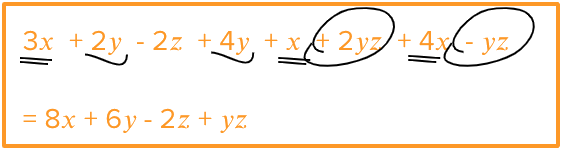# Addition and Subtraction of Algebraic Expressions

Go back to  'Algebra Basics'

## What are like and unlike terms in algebra?

Take a look at these terms:

 $$7x \qquad \qquad x \qquad \qquad −2x \qquad \qquad 13x \qquad \qquad 4x$$ The variable in each term is $$x.$$

Now look at these terms:

 $$3xy \qquad \qquad −3y \qquad \qquad 12y^3 \qquad \qquad −8x^2 \qquad \qquad y^2 \qquad \qquad xy^2$$ All of these terms have different variables. No two terms have the same variable

When the variable of terms is the same, those terms are called like terms.

If the variables in the two terms are different, then those two terms are called, unlike terms.

## Adding and Subtracting in Algebra

 Only like terms can be combined by adding or subtracting them with one another. Unlike terms cannot be combined by adding or subtracting.

Think of adding and subtracting in algebra in two ways:

1. You have one algebraic expression. It can be simplified by adding and subtracting like terms within it.
2. You have two algebraic expressions and are asked to add or subtract them. After adding or subtracting, you’ll end with one final algebraic expression.

Let’s look at both scenarios.

## Adding and Subtracting Like Terms in an Expression

Look at this expression:

$6x^2 + 7x + 8x^2 + 9 + 8y$

Observe the variables of each term. You’ll see that there are two terms with variable $$x^2.$$

$6x^2 + 7x + 8x^2 + 9 + 8y$

That makes $$6x^2$$ and $$8x^2$$ like terms. They can be added or subtracted with each other.

 When adding or subtracting like terms, the coefficients are added (or subtracted). The variable remains unchanged.

So, the expression above will simplify to:

$14x^2 + 7x + 9 + 8y$

Be careful with the signs. If a term has a negative sign before it, consider it while adding/subtracting.

$2x − 5xy − 11 + 2xy + y^2$

Here the $$xy$$ terms are like terms. The constant terms are also like terms (neither have a variable). So on simplification, the expression becomes:

$2x − 3xy − 8 + y^2$

## Adding and Subtracting Two Algebraic Expressions

At times you will have two algebraic expressions that you will have to add or subtract.

 $(p + 2q + 3r + 4) + (2p + 4q + 6r + 2)$ To do this, simply open out the brackets like we do with whole numbers and then add or subtract. Step 1: Open out the brackets $p + 2q + 3r + 4 + 2p + 4q + 6r + 2$ Step 2: Combine the like terms to get the simplified expression $3p + 6q + 9r + 6$

Be careful when subtracting:

 $(6a + 2b-3c) - (4a-4b + 9c + 12)$ When opening a bracket when there’s a subtraction sign before it, this sign will flip the sign of all terms inside the bracket. Step 1: Open out the brackets $6a + 2b-3c-4a + 4b-9c-12$ Step 2: Combine the like terms to get the simplified expression $2a + 6b - 12c - 12$

## Tips and Tricks to Add and Subtract Algebraic Expressions

• A useful technique when simplifying expressions is to use different patterns of underlining for different sets of like terms. This makes it easy to add and subtract.• When adding expressions, children who are just learning algebra often tend to think that “$$3x + 5y$$” equals “$$8xy$$”. Watch out for this misconception. Revise the concept of like and unlike terms by asking them to form expression using everyday situations. Help children see why only like terms can be added this way.
• Tip: Give targeted practice involving addition and subtraction of expression where there is a minus sign just before a bracket:
$$3 - (y - 2)$$
Children may incorrectly simplify this as $$3 - y - 2 = 1 - y$$

Instead the correct simplification will be $$3 - y + 2 = 5 - y$$